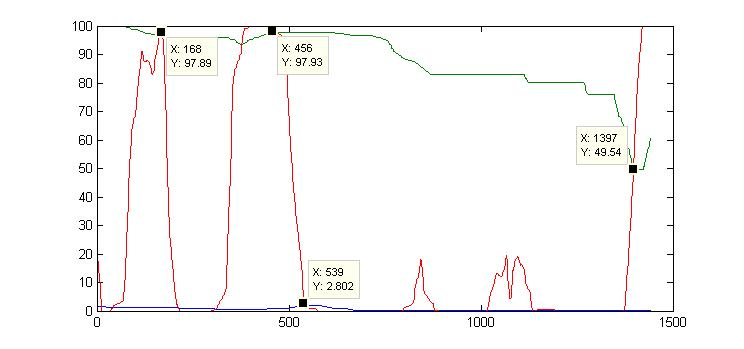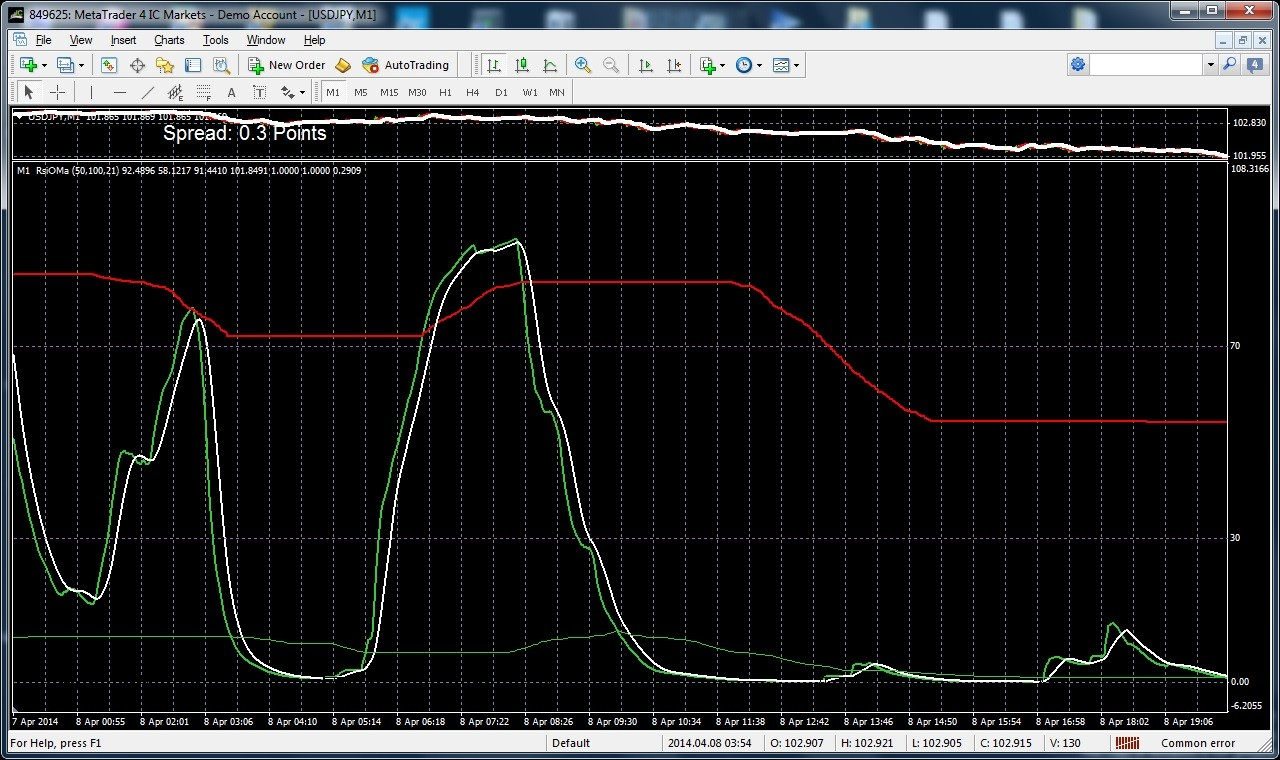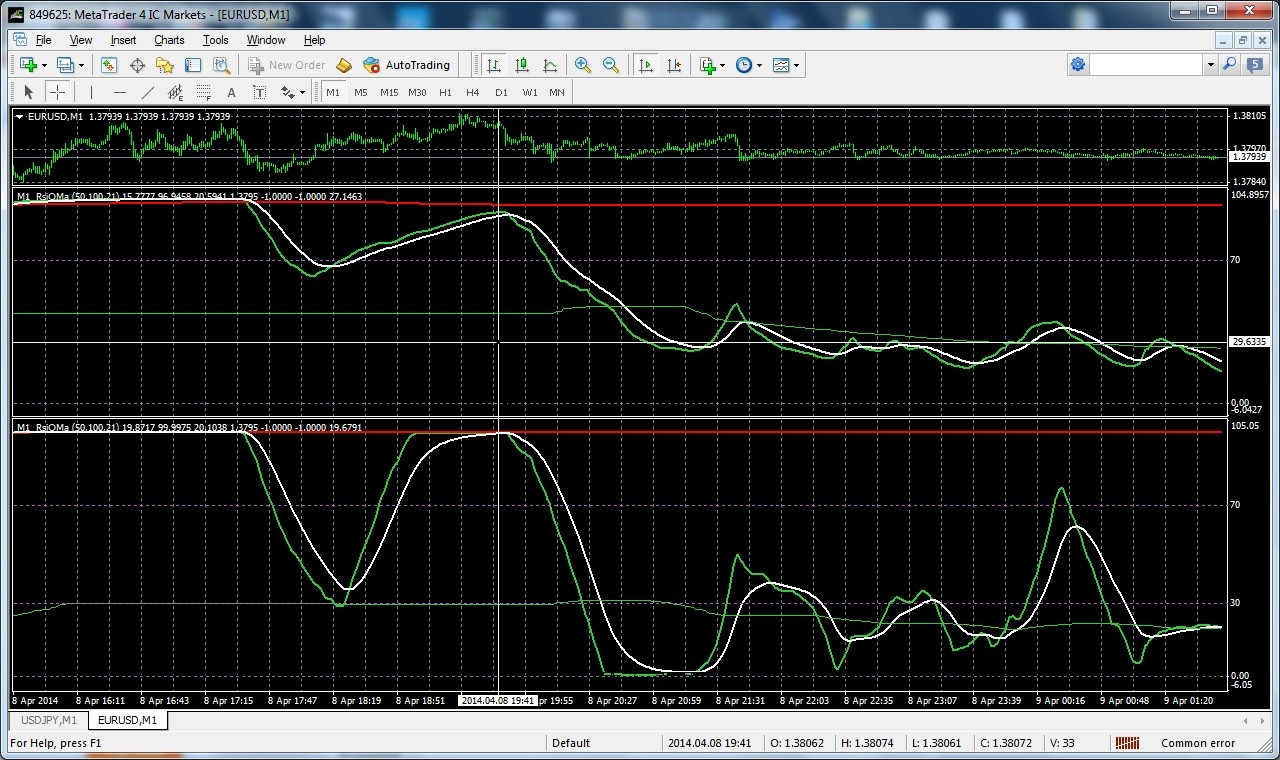# RSI of Moving Average - page 32155996

fajst_k:
I think OHLC is held. I just made another test and if you plot simple RSI mismatch don't occur.

(basic test methodology - if nothing works back to the square 1). So RSI which is implemented

in MT4 as separate script is working.

But i think during 'test mode' some imprecisions are occuring most likely for speeding up as the biggest mismatch occurs for this test in detrended mode as Close-mab is a very small value.

So all those iMAOnArray/iRSIOnArray/iMA internally may use much lower precision than 'double'

Simple test. Rewrite this indi using scripts like SimpleMAOnBuffer from MovingAverages.mqh

but not build in functions would confirm/deny this theory

Their SimpleMAOnBuffer has the same error as their iMAOnArray (they use the same way of calculating). It is the way that is made to be faster but they overlooked the case of repeating values and SMA.

Here is a function that always calculates correct values, and is as fast as that fast way they are using (even in those cases of repeated values, so all in all, it was just a matter of writing it using different calcuation way and predicting what can happen with the way they are using):

double workSma[];

double iSma(double price, int period, int r, int instanceNo=0)

{

if (ArrayRange(workSma,0)!= Bars) ArrayResize(workSma,Bars); r = Bars-r-1; int k = period; instanceNo *= 2;

workSma[r] = price;

if (r>=period)

workSma[r] = workSma[r-1]+workSma[r]-workSma[r-period];

else { workSma[r] = 0; for(k=0; k=0; k++) workSma[r] += workSma[r-k]; }

return(workSma[r]/k);

}298

Their SimpleMAOnBuffer has the same error as their iMAOnArray (they use the same way of calculating). It is the way that is made to be faster but they overlooked the case of repeating values and SMA.

Here is a function that always calculates correct values, and is as fast as that fast way they are using (even in those cases of repeated values, so all in all, it was just a matter of writing it using different calcuation way and predicting what can happen with the way they are using):

double workSma[];

double iSma(double price, int period, int r, int instanceNo=0)

{

if (ArrayRange(workSma,0)!= Bars) ArrayResize(workSma,Bars); int k = period; instanceNo *= 2;

workSma[r] = price;

if (r>=period)

workSma[r] = workSma[r-1]+workSma[r]-workSma[r-period];

else { workSma[r] = 0; for(k=0; k=0; k++) workSma[r] += workSma[r-k]; }

return(workSma[r]/k);

}

Thanks. I ve seen this function in a lot of your indicators, however didn't know why is there...now i know. Anyway this bug can explain a lot of problems with MT4 tester, I'm just curious how much money traders lost due to this bug...

Krzysztof992

Their SimpleMAOnBuffer has the same error as their iMAOnArray (they use the same way of calculating). It is the way that is made to be faster but they overlooked the case of repeating values and SMA.

Here is a function that always calculates correct values, and is as fast as that fast way they are using (even in those cases of repeated values, so all in all, it was just a matter of writing it using different calcuation way and predicting what can happen with the way they are using):

double workSma[];

double iSma(double price, int period, int r, int instanceNo=0)

{

if (ArrayRange(workSma,0)!= Bars) ArrayResize(workSma,Bars); int k = period; instanceNo *= 2;

workSma[r] = price;

if (r>=period)

workSma[r] = workSma[r-1]+workSma[r]-workSma[r-period];

else { workSma[r] = 0; for(k=0; k=0; k++) workSma[r] += workSma[r-k]; }

return(workSma[r]/k);

}

Nice

Tested it on some data and it really fives correct results298

RSOMA non detrended results

Here are results for RSIOMA EA non detrend setting for 6 pairs for 1 week 1 min data. Seems to be slightly profitable

Krzysztof

USDJPY Gross profit 67.74 Gross loss -85.46

GBPUSD Gross profit 118.30 Gross loss -133.40

GBPJPY Gross profit 127.93 Gross loss -217.59

EURUSD Gross profit 194.50 Gross loss -77.30

AUDUSD Gross profit 126.80 Gross loss -97.50

AUDJPY Gross profit 163.33 Gross loss -126.12

PF 1.08

Files:298

RSOMA detrended results

and here are some RSOMA results with detrending.

USDJPY Gross profit 204.17 Gross loss -124.32

GBPUSD Gross profit 98.10 Gross loss -126.90

GBPJPY Gross profit 285.54 Gross loss -250.14

EURUSD Gross profit 135.30 Gross loss -159.70

AUDUSD Gross profit 135.40 Gross loss -127.30

AUDJPY Gross profit 119.84 Gross loss -289.95

PF = 0.9

Seems detrending makes more harm than good

Files:
detrended.rar 52 kb298

I cloned RSIOMA with DZ to MATLAB and discovered another surprise. Here is a screenshot of MATLAB plotFiles:
rsioma_1.jpg 25 kb298

and here screenshoot from MT4 on the same data with the same settings and its clear difference between them. The timing of crosses

between RSI and DZ seems to be similar, overall shape also but absolute values of RSI and DZ very different !!! Only explanation of it is that RSI calculated as iRSIOnArray is calculated in some strange way like e.g. internal smoothing is applied which supresses absolute levels of RSI. Can someone explain this ??

KrzysztofFiles:
rsiomamt4.jpg 324 kb298

here is a screenshoot of RSIOMA based on Wilder RSI (the most bottom window) and MT4 RSI. Clearly the slopes of Wilder

RSI are more horizontal so Dynamic zones are much wider.Files:
wilderrsi.jpg 358 kb298

and the code of function which calculates Wilder RSI

double rrsi(double& data[], int period, int j)

{

int i;

double diff;

double declines;

double rs;

double rrsi;

for(i=j; i<=j+period; i++)

{

diff=data-data;

if(diff>=0)

else

declines-=diff;

}

// Calculate RSI

if (declines !=0){

rrsi = 100 - (100 / (1+rs));

}

else rrsi = 100;

return rrsi;

}155996

Krzysztof

You might want to read this post : https://www.mql5.com/en/forum/183208/page2

It might save you some time (I was looking for my coding error for 1 whole day only to find out that the error was not in my code at all)

regards